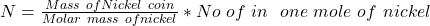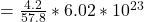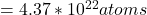## We want to find how much charge is on the electrons in a nickel coin. Follow this method. A nickel coin has a mass of about 4.2 g. Find the

Question

We want to find how much charge is on the electrons in a nickel coin. Follow this method. A nickel coin has a mass of about 4.2 g. Find the number of atoms in a nickel coin. Each mole (6.02 × 1023 atoms) has a mass of about 57.8 g. Answer in units of atoms

in progress 0
1 month 2021-08-12T07:58:31+00:00 1 Answers 1 views 0

The number of atoms is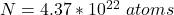Explanation:

From the question we are told that

The mass of coin  is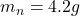Number of atom in one mole =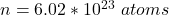Molar mass of nickel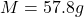Now the relation to obtain the number of atom in  the  nickel coin is